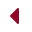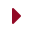## Contact

Last Updated on

Information made available to us through the contact form is subject to the terms and conditions outlined in the disclaimer. Please take some time to go through it.

Feedback is automatically filtered for spam and unsolicited offers, so all you “white hat SEO optimizers” better not bother contacting me.

## Mailbag

Cabal Times receives a variety of interesting mail and feedback. Some are outright genius. Some are loopy. But like the readers of this website, almost all feedback is unique and eccentric. The following are some selections.

New Revelations About the Nature of Time or Crackpot? Sent by Duane Ertle

Dangerous Ideas © 2011, D. Ertle The enclosed is information that may be of interest to you. It has been sent to different nations in expectqation they will act upon the information, taking it at face value till proven either right or wrong. There is a manner by which the enclosed writing is able to be proved to be correct, which is to perform a gravitational experiment. I have done this by means of a twelve-foot long beam, and a two-inch beam, having a lead weight attached to one side of it. I used a laser to determing beam movement when using the two-inch beam. At “youtube.com”, under the tltle “Successful Gravity Experiment”, done by “Stcorridon”, an experiment has been posted showing the increase and decrease of a field of gravity. Dear Sir, I am writing and sending this to you because I wish asylum from the United States of America to that of another nation. My complaint extends over the duration of 30+ years. After reading this, and should you have an interest, there is a posting at “bonesoftime.wordpress.com” under the science category that gives an outline of the 30 years. It is not exhaustive. The reason the U.S. wishes to suppress what I have done is my insistence that evolution is a delusion (we are too complex, and time ‘entropy’ destroys, only. Time does not form complex design). Also, I maintain that abortion is murder, and the ‘big bang’ an impossibility. The basic beliefs that I espouse are very politically incorrect. I wish to speak in particular about physical time. I was here that the science work I have done began. It starts with the physics trilogy, which is: m=E/c^2, E=mc^2, and c^2=E/m. The last equation, which completes the trilogy, describes a field of physical time. It also describes why a field of gravity exists. I’ll begin with the time value itself. Notice that in each of these three equations that the mass and energy values may change while that of c^2 does not change. The reason for this is that our universe is wholly and totally composed of time. It is for this reason all clocks are able to relate to one another in an exact same manner. The value of ‘c’ exists as the formation of all time standards. Whether in the movement of the stars and planets or the vibrations of an atomic clock, everything relates to that which it is formed of. The equation c^2=E/m also describes how a field of gravity forms. Notice that it is a mass/energy arrangement. As the heat energy increases within a mass so also does the intensity of the gravitational field. This means the value that we call the “gravitational constant” is not a constant at all, but is a variable. Were the heat energy within our planet to increase while the mass remained the same, then earth’s field of gravity would increase proportionally. Were the mass of our planet to have no heat energy within it, then our planet would have no gravity. Apart from an energy value, the equation c^2=E/m would become “m”. c^2=E/m is the general equation for a field of physical time while the equation c=h is the specific equation. c=h describes the value of ‘c’ as having two values, that of both speed and energy. c=h is formed of two constants, each having a value per second. This equation describes either ‘c’ or ‘h’ as being similar to a thrown baseball that has both velocity and energy; energy being able to be determined by velocity and velocity by its energy. c=h is the specific value for time/gravity, and is the value of individual gravitons. The value for c=h is found according to c^2xh, this determines the shortest distance for ‘c’. The answer is c^2xh=5.955329639 e -17 meter. This is the value we seek to reduce ‘c’ (299,792,500 meters) to. After reducing ‘c’ 67 times by 0.5, the distance value for ‘c’ is near an electron wavelength (approximately 2.031472712 e -12 meter). It requires 15 more reductions by 0.5 to arrive at the value we seek, which demonstrates the shortness/smallness of physical time. The final result is a distance of 6.199563594 e -17 meter Because distance is reduced 82 times, so also is a second to 1.986483864 e -25 second, and so also is the value of energy ‘h’, to 1.370265216 e -58 Joule. This last value describes the small energy of individual gravitons, which is why they cannot be captured. Physical time at this juncture is able to be described as c=h becoming: (t=1 = h=1), each having the value of h=1. (t=1 = h=1) becomes: time without energy and energy without time. Lastly, regarding c=h, is the fact that this small value is the basis of all physical existence. It is the answer to the Theory of Everything. Because c=h is “time” itself, this becomes the specific meaning of it. Our universe is formed of time according to the general equation c^2=E/m, meaning there is no mass or energy not totally formed of the value c=h. c=h describes what we call ‘the passage of time”, it moving at a speed of 6.199563594 e -17 meter during the duration of 1.986483864 e -25 second, and having an energy value of 1.370265216 e -58 Joule per c=h. All creation moves this spatial distance during this time span, which is why reality has a past, present, and future. Eleven Year Sun Cycles This short writing has to do with our sun having eleven year sun cycles when forming the greatest number of sun spots, the answer as to why this happens becoming evident by the asking of four question. The basis of these questions is in the recognition that c2=E/m describes a field of time, commonly understood to be that of gravity. First, what would happen to our sun if there were to be an increase of heat energy (E) within its mass? The answer is, the field of time/gravity about our sun would increase due to a greater energy to mass ratio. The second question is, what would happen if all the heat energy within our sun were to vanish, leaving mass only? The answer is, there would be no gravitational field about our sun because there would exist no energy (E) to form it. As energy within a mass decreases so does the field of gravity. Third, what would happen if the sun’s mass were to increase? Were this to occur, there then would be an increase of gravity. Greater mass would cause the overall density of our sun to increase due to greater mass being drawn downward toward its center. This in turn would compress the existing mass, heating it to a greater extent, causing an increase of gravity. The fourth question is obvious, as is the answer to the question. What would happen if our sun would have a decrease of its mass? This is its condition at present. Our sun is continually losing mass by reason of radiant energy being emitted, loss of atomic particles that form the solar wind, and the dissipation of its field of gravity (665 lbs/sec). What does this loss of mass mean? As an increase of mass causes an increase of a field of gravity, the decrease of mass causes a decrease of this force. As the “pressure” of downward mass within our sun decreases, the mass surrounding the greatest gravity value must also decrease in density. It does this by moving outward from the center of our sun. As it moves outward, due to a decreasing gravitational field, the mass carries with it internal heat energy, cooling the interior of the sun just a little. It is this energy rising to the sun’s surface in a burst of sudden adjustment of intensity that gives rise to sunspots. Every eleven years our sun forms a new energy baseline where there is an energy to mass gravitational balance, when there exists a period of solar rest. After a lapse of quiet years there forms a new adjustment of solar mass, excess energy once again is released and the cycle begins anew. Because of an increase of sunspot energy averages moving upward, it appears our sun could be headed toward becoming a nova, when a major mass/energy release begins (similar to earth tremors leading to a major earthquake). It’s all about time.